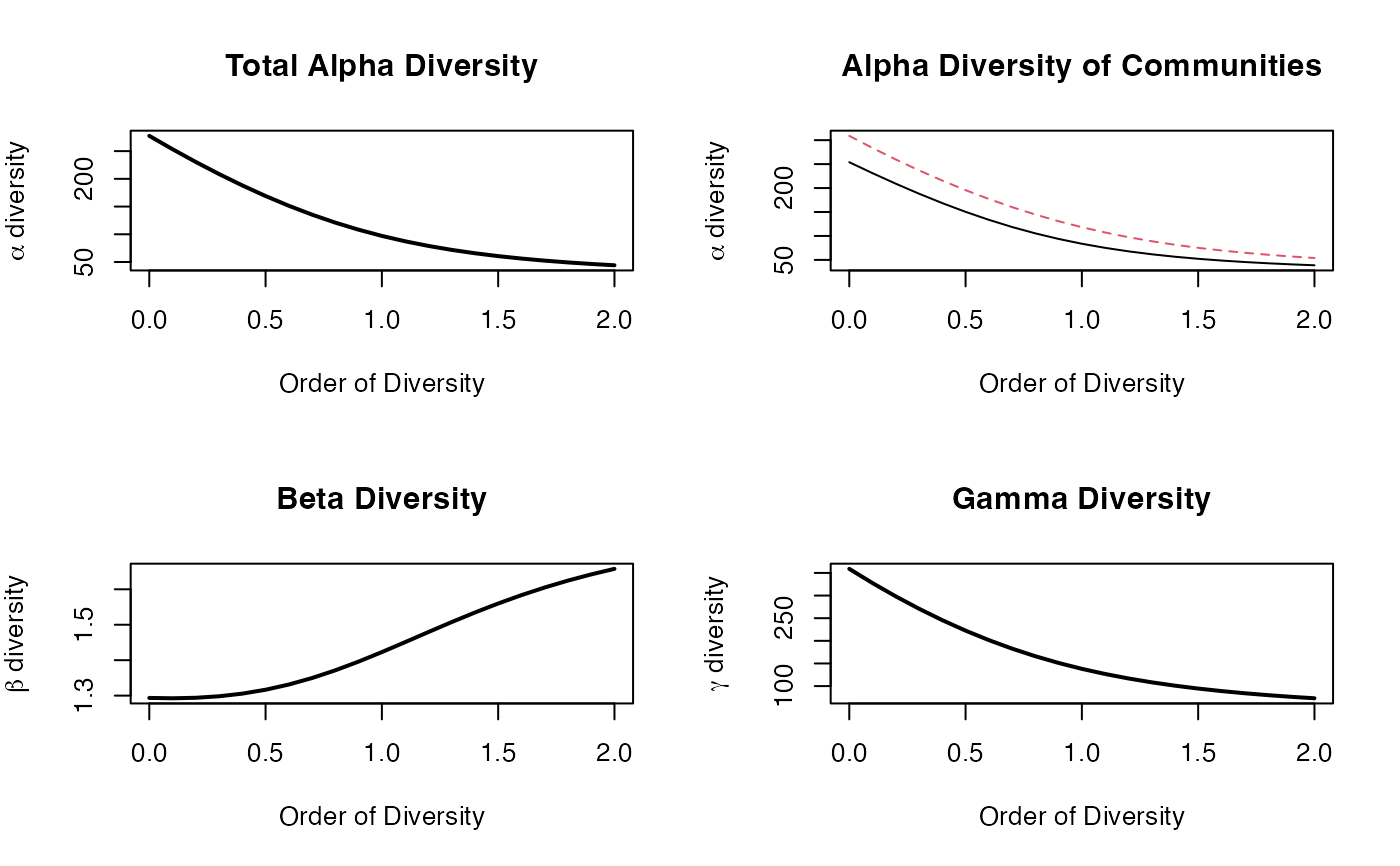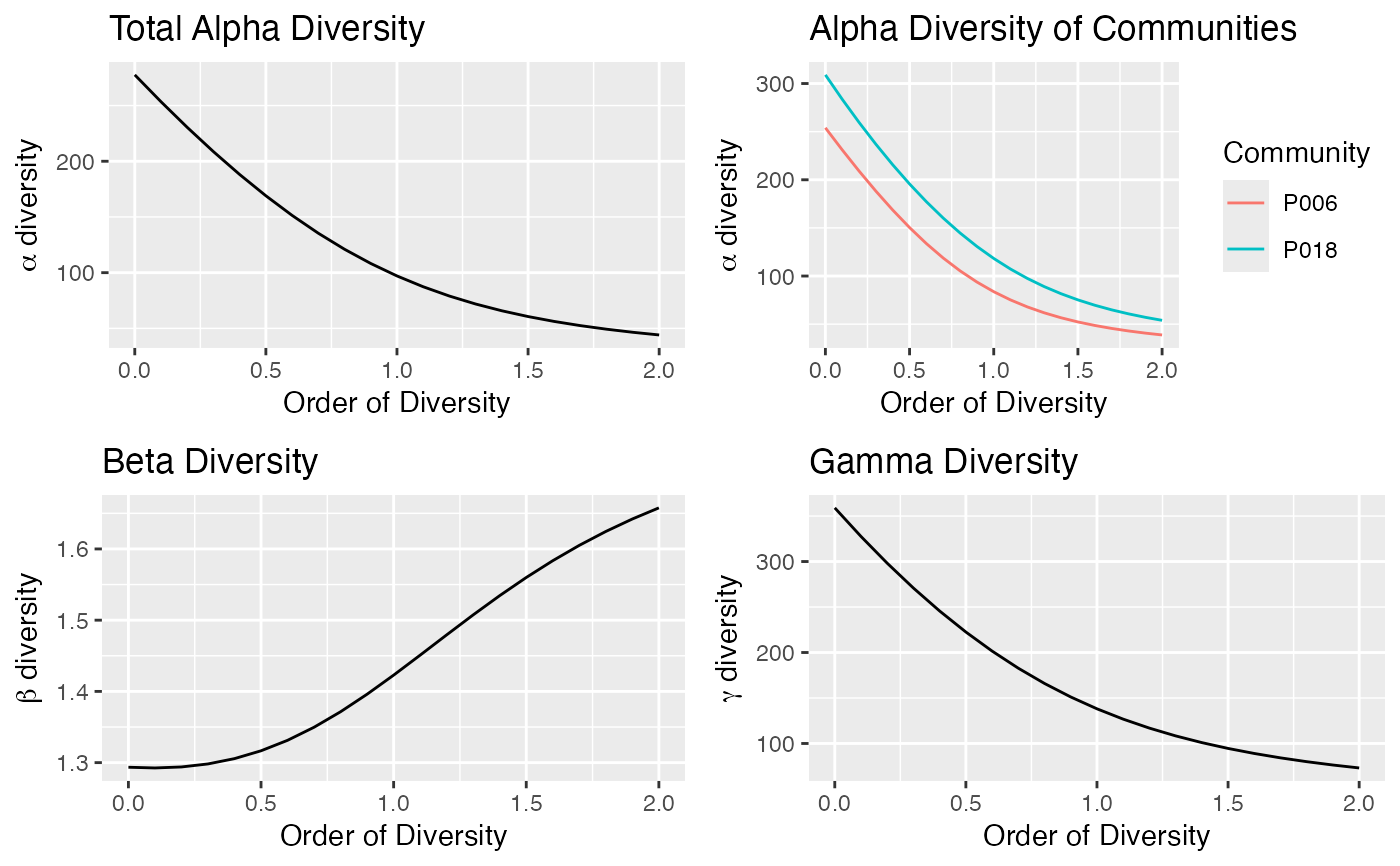Calculate the diversity profiles (alpha, beta, gamma) of a metacommunity.

DivProfile(q.seq = seq(0, 2, 0.1), MC, Biased = TRUE, Correction = "Best",
Tree = NULL, Normalize = TRUE, Z = NULL,
NumberOfSimulations = 0, Alpha = 0.05,
ShowProgressBar = TRUE, CheckArguments = TRUE)
is.DivProfile(x)
# S3 method for DivProfile
plot(x, ..., main = NULL, xlab = "Order of Diversity",
ylab = NULL, Which = "All",
LineWidth = 2, ShadeColor = "grey75", BorderColor = "red")
# S3 method for DivProfile
autoplot(object, ..., main = NULL, xlab = "Order of Diversity",
ylab = NULL, Which = "All", ShadeColor = "grey75", alpha = 0.3, BorderColor = "red",
labels = NULL, font.label = list(size=11, face="plain"),
col = ggplot2::GeomLine$default_aes$colour,
lty = ggplot2::GeomLine$default_aes$linetype,
lwd = ggplot2::GeomLine$default_aes$size)
# S3 method for DivProfile
summary(object, ...)

## Arguments

q.seq

A numeric vector.

MC

A MetaCommunity object.

Biased

Logical; if FALSE, a bias correction is appplied.

Correction

A string containing one of the possible corrections.

The correction must be accepted by AlphaEntropy, BetaEntropy and GammaEntropy. "Best" is the default value.

Tree

An object of class hclust, phylo, phylog or PPtree. The tree must be ultrametric.

Normalize

If TRUE (default), diversity is not affected by the height of the tree.
If FALSE, diversity is proportional to the height of the tree.

Z

A relatedness matrix, i.e. a square matrix whose terms are all positive, strictly positive on the diagonal. Generally, the matrix is a similarity matrix, i.e. the diagonal terms equal 1 and other terms are between 0 and 1.

NumberOfSimulations

The number of simulations to run, 0 by default.

Alpha

The risk level, 5% by default.

ShowProgressBar

If TRUE (default), a progress bar is shown.

CheckArguments

Logical; if TRUE, the function arguments are verified. Should be set to FALSE to save time when the arguments have been checked elsewhere.

x

An object to be tested or plotted.

main

The main title of the plot. Ignored if Which = "All".

xlab

The x axis label of the plots.

ylab

The y axis label of the plot. Ignored if Which = "All".

Which

May be "Communities", "Alpha", "Beta" or "Gamma" to respectively plot the alpha diversity of communities or the metacommunity's alpha, beta or gamma diversity. If "All" (default), all four plots are shown.

LineWidth

The width of the line that represents the actual profile.

The color of the shaded confidence envelope.

BorderColor

The color of the bounds of the confidence envelope.

alpha

Opacity of the confidence enveloppe, between 0 and 1.

labels

Vector of labels to be added to multiple plots. "auto" is the same as c("a", "b", "c", "d)".

font.label

A list of arguments to customize labels. See ggarrange.

col

The color of the geom objects. See "Color Specification" in par.

lty

The type of the lines. See lines.

lwd

The width of the lines. See lines.

object

A MCdiversity object to be summarized or plotted.

...

Additional arguments to be passed to the generic methods.

## Details

If Tree is provided, the phylogenetic diversity is calculated.

DivPart partitions the diversity of the metacommunity into alpha and beta components. It supports estimation-bias correction.

If Tree is provided, the phylogenetic diversity is calculated else if Z is not NULL, then similarity-based entropy is calculated.

Beta diversity/entropy is calculated from Gamma and Alpha when bias correction is required, so community values are not available.

If NumberOfSimulations is greater than 0, a bootstrap confidence interval is produced by simulating communities from a multinomial distribution following the observed frequencies (Marcon et al, 2012; 2014) and calculating their profiles.

## Value

A DivProfile object. It is a list:

MetaCommunity

The name of the MetaCommunity object containing inventory data.

Order

A vector containing the values of q.

Biased

Logical. If FALSE, bias corrected values of diversity have been computed.

Correction

The estimation bias correction used to calculate diversity. Usually a string, but it may be a list if different corrections have been used in the estimation of phylodiversity.

Method

The method used to calculate entropy ("HCDT", "Similarity-based").

Tree

The phylogenetic or functional tree used to calculate phylodiversity.

Normalized

Logical. Indicates whether phylodiversity is normalized or proportional to the height of the tree.

Z

The matrix used to calculate similarity-based entropy.

A matrix containing the alpha diversity of each community.

A vector containing the alpha diversity of communities for each order.

A vector containing the beta diversity of communities for each order.

A vector containing the gamma diversity of the metacommunity for each order.

CommunityAlphaEntropies

A matrix containing the alpha entropy of each community.

TotalAlphaEntropy

A vector containing the alpha entropy of communities for each order.

BetaEntropy

A vector containing the beta entropy of communities for each order.

GammaEntropy

A vector containing the gamma entropy of the metacommunity for each order.

Confidence envelopes

Total Alpha, Beta and Gamma Entropy and Diversity may come with a confidence envelope whose value is stored in twelve more vectors named suffixed Low or High, such as GammaEntropyLow

DivProfile objects can be summarized and plotted.

## Author

Eric Marcon <Eric.Marcon@agroparistech.fr>, Bruno Herault <Bruno.Herault@cirad.fr>

DivPart

## Examples

# Load Paracou data (number of trees per species in two 1-ha plot of a tropical forest)
data(Paracou618)
# Estimate diversity.
Profile <- DivProfile(q.seq = seq(0, 2, 0.1), Paracou618.MC, Biased = FALSE)
plot(Profile)autoplot(Profile)summary(Profile)
#> Diversity profile of MetaCommunity Paracou618.MC
#>  with correction: UnveilJ
#> Diversity against its order:
#>         Order Alpha Diversity Beta Diversity Gamma Diversity
#> UnveilJ   0.0       277.53648       1.293524       359.00000
#> UnveilJ   0.1       253.43317       1.292524       327.56839
#> UnveilJ   0.2       230.43524       1.293933       298.16776
#> UnveilJ   0.3       208.62940       1.298170       270.83648
#> UnveilJ   0.4       188.11846       1.305629       245.61294
#> UnveilJ   0.5       169.01163       1.316623       222.52453
#> UnveilJ   0.6       151.41091       1.331320       201.57635
#> UnveilJ   0.7       135.39585       1.349686       182.74194
#> UnveilJ   0.8       121.00956       1.371445       165.95797
#> UnveilJ   0.9       108.24942       1.396073       151.12409
#> UnveilJ   1.0        97.06467       1.422843       138.10783
#> UnveilJ   1.1        87.36143       1.450904       126.75305
#> UnveilJ   1.2        79.01317       1.479377       116.89026
#> UnveilJ   1.3        71.87395       1.507453       108.34662
#> UnveilJ   1.4        65.79132       1.534463       100.95435
#> UnveilJ   1.5        60.61669       1.559914        94.55679
#> UnveilJ   1.6        56.21256       1.583490        89.01206
#> UnveilJ   1.7        52.45645       1.605040        84.19469
#> UnveilJ   1.8        49.24224       1.624534        79.99569
#> UnveilJ   1.9        46.47987       1.642037        76.32167
#> UnveilJ   2.0        44.09399       1.657672        73.09335